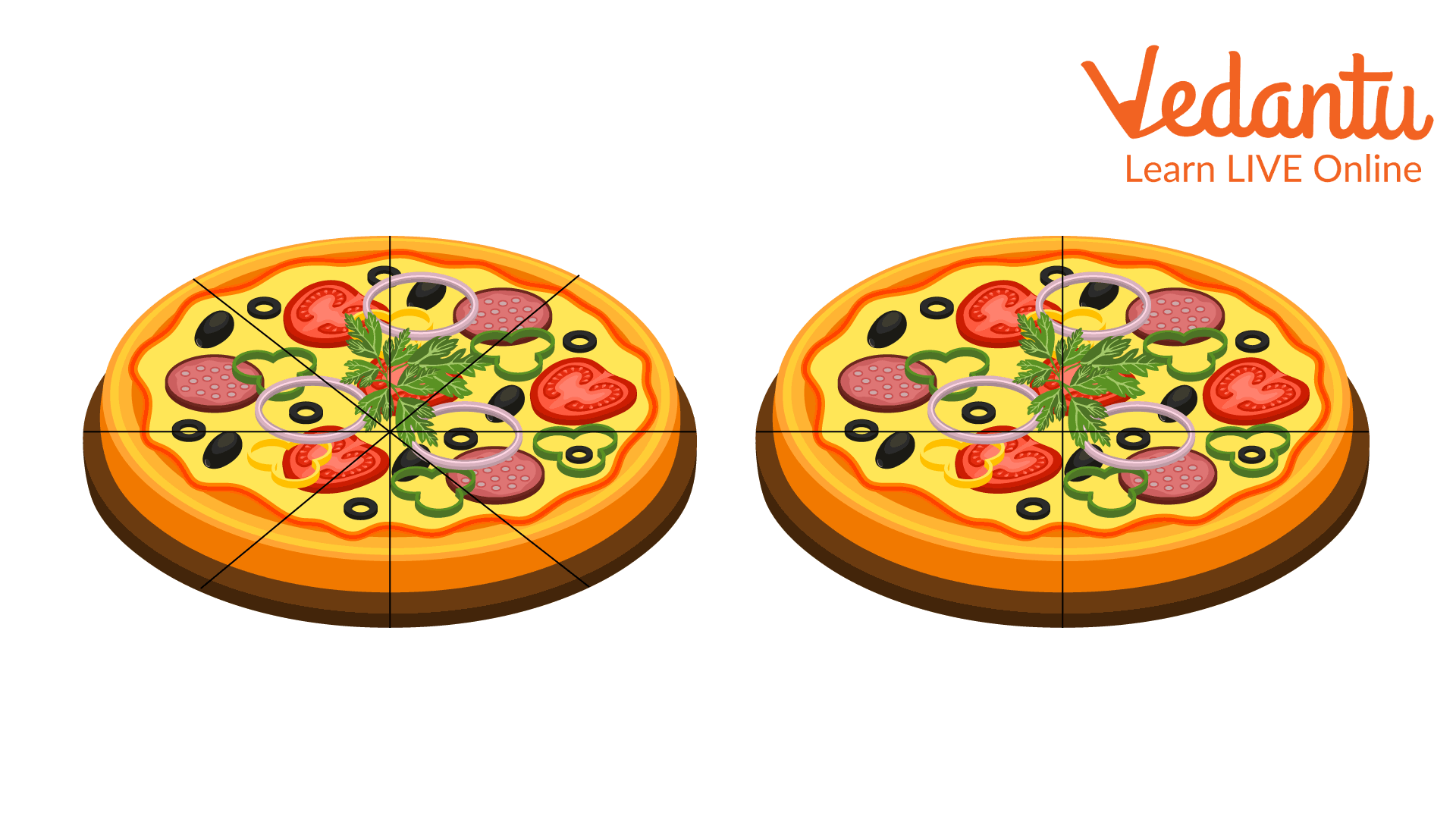Courses
Courses for Kids
Free study material
Offline Centres
More

# Proportion ProblemsLast updated date: 05th Dec 2023
Total views: 99.6k
Views today: 0.99k## How to Solve Proportion Problems?

Have You Ever Wondered What Proportions Are?
Imagine you have a pizza cut into 8 pieces; of these 8 pieces, you can only eat 2 before you are full. Now, the next day you eat another pizza cut into 4 pieces, wherein you can eat only 1 of the pieces before your stomach is full. How do you determine if you ate the same amount on both days? Here is where proportion comes in. It is used when you have to compare two fractions.Pizza sliced in 8 and 4 pieces to show the proportion

## How Are Proportions Written?

Proportions can be written in two ways:

1. $\frac{a}{b}:\frac{c}{d}$

2. $a:b::c:d$

In both these instances, ‘a’ and ‘d’ are the extremes while ‘b’ and ‘c’ are known as the middle terms or the means.

## How to Solve Proportion Problems?

There is one simple rule to check if the two fractions are in proportion,

Product of the middle terms = Product of the Extremes

$b\times c=a\times d$

If $b\times c\ne a\times d$, the two given fractions are not in proportion.

## Examples of Proportion Problems

Q1. Determine which of these is in proportion. Fill in the blank with either an equal to sign $=$ or a not equal sign $\ne$.

1. $\frac{8}{18}$ $\frac{1}{9}$

1. $\frac{4}{12}$ $\frac{9}{27}$

Solutions:

1. $\frac{8}{18}$ $\frac{1}{9}$

This can be written as $8:18::1:9$which is in the format $a:b::c:d$

On solving, we get,

Product of means = $18\times 1=18$

Product of extremes = $8\times 9=72$

Since $18\ne, 9$the two ratios are not in proportion.

Ans: $\frac{8}{18}\ne \frac{1}{9}$

1. $\frac{4}{12}$ $\frac{9}{27}$

This can be written as $4:12::9:27$which is in the format $a:b::c:d$

On solving, we get,

Product of means = $12\times 9=108$

Product of extremes = $4\times 27=108$

Since $108=108$the two ratios are in proportion.

Ans: $\frac{4}{12}=\frac{9}{27}$

Q2. Complete the following proportions

1. $1:3::4:\_$

2. $5:2::\_:10$

Solutions:

1. Since $1:3::4:\_$is already in the format of $a:b::c:d$we can directly equate the products and solve for ‘d’

$b\times c=a\times d$

$3\times 4=1\times d$

∴$d=12$

Ans: 12

1. Since $5:2::\_:10$is already in the required format, we must now solve for ‘c’

$2\times c=5\times 10$

$c=\frac{50}{2}$

∴$c=25$

Ans: 25

Q3. One boy eats 12 out of 15 slices of pizza. How many slices of pizza should another boy eat if he has a pizza with 5 slices and wants to eat the same amount as the first boy?

Solution:

To solve a word problem, first, you must take the given data and make it into ratios.

The first boy ate 12 out of 15, so we have $\frac{12}{15}$

The second boy has a total of 5 slices, so we must find $\frac{c}{5}$

This gives us the ratios in the following format $\frac{a}{b}:\frac{c}{d}$

We know, $b\times c=a\times d$

$15\times c=12\times 5$

∴$c=\frac{12\times 5}{15}$

∴$c=4$

Ans: The second boy should eat 4 slices of pizza.

Q4. If $a:b=3:7$and $b:c=5:9$then, find $a:c$

Solution:

We know that ratios can be written as fractions

So $a:b=\frac{a}{b}$ and $b:c=\frac{b}{c}$

∴ $\frac{a}{b}\times \frac{b}{c}=\frac{a}{c}$

∴$\frac{3}{7}\times \frac{5}{9}$ = $\frac{5}{21}$

Ans: $a:c=5:21$

Q5. If $a:b=3:7$and $b:c=5:9$then, find $a:b:c$

Solution:

To find this ratio, the value of ‘b’ must be the same in both ratios. The proportion remains the same when a fraction is multiplied by the same number in the numerator and denominator.

To make the value of ‘b’ equal in both these fractions, we must multiply the first by 5 and the second by 7.

$\frac{3\times 5}{7\times 5}$ and $\frac{5\times 7}{9\times 7}$

= $\frac{15}{35}$ and $\frac{35}{63}$making the value of ‘b’ equal

We can therefore write the equation $a:b:c=15:35:63$

## FAQs on Proportion Problems

1. What are direct proportions?

When two ratios or fractions are proportional to each other, and their values are constant, they are said to be directly proportional to each other or direct proportions.

2. How many types of proportions exist?

Proportions are of four types

• Direct Proportions

• Inverse Proportions

• Compound Proportions

• Continued Proportions

3. Can all ratios be considered proportions?

No. Ratios are a comparison between two quantities or items, whereas a proportion is a comparison between two ratios. We can say that a ratio is an expression while a proportion is an equation, the key difference being the ‘equal to’ sign we use while determining proportions.